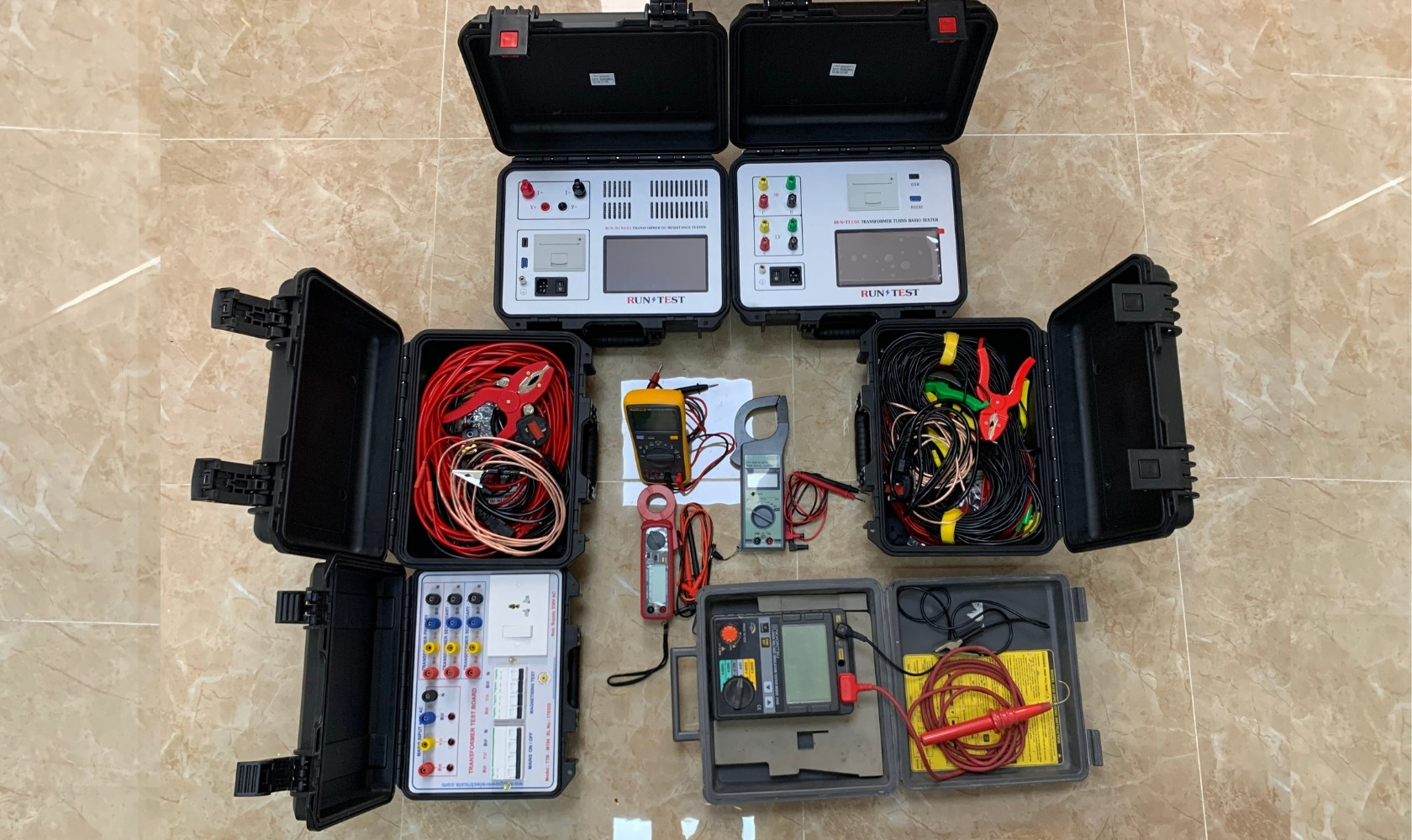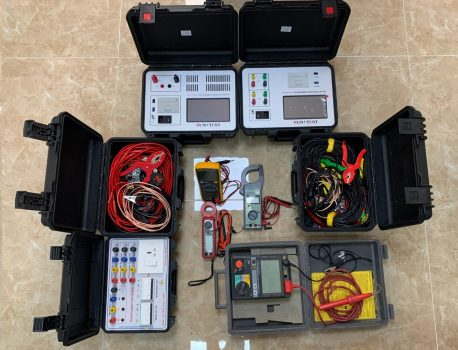# Testing### Testing

We do on-site testing of transformers, Shunt reactors and Generators

### Transformer Routine Test

• Transformer turns ratio
• Winding resistance
• Magnetic balance
• Measurement Magnetizing current
• Vector Group test
• Insulation Resistance
• Dielectric Break down Voltage test for Oil (Oil BDV)

### Transformer Special Test

• Sweep Frequency Response Analysis (SFRA)
• Transformer Tan Delta & Capacitance Measurement
• Bushing Tan Delta & Capacitance measurement
• On-line Partial Discharge (PD) measurement with Aquatic method

### Current Transformer Test

• Insulation Resistance
• Ratio test
• Winding resistance
• Polarity
• Knee point measurement

### Shunt Reactor Test

• Insulation Resistance
• Winding Resistance
• Tan Delta & Capacitance measurement
• Current transformer testing